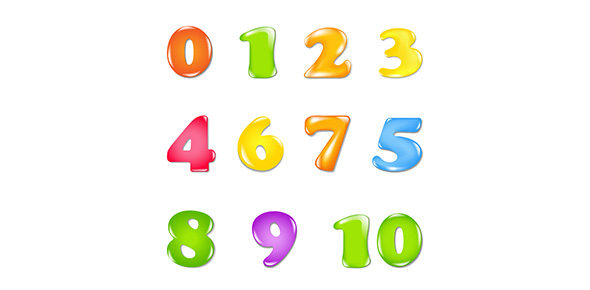# Binary Number And Code Systems Quiz!

10 Questions | Attempts: 1583SettingsThe binary number system is a numbering system that indicates numeric values using two peculiar digits 0 and 1. Mostly all computer devices use the binary numbering system to represent electronic circuit voltage state. This quiz has been created to test your knowledge about the correct binary codes and numbers representation. So, let's try out the quiz. All the best!

• 1.
What is the binary representation for 0xAC ?
• A.

10101001

• B.

10101010

• C.

10101100

• D.

11001010

• E.

11111111

• 2.
What is the hexadecimal representation for the binary number 100100101011 ?
• A.

0xABC

• B.

0x911

• C.

0x92B

• D.

0x92C

• E.

0x94C

• 3.
What is the base 10 number 233, expressed as binary ?
• A.

11101001

• B.

11111001

• C.

11101011

• D.

11011011

• E.

None of the above

• 4.
What is the base 10 number, 312, expressed as hexadecimal ?
• A.

0xCC2

• B.

0x3B2

• C.

0x444

• D.

0x138

• E.

0xEF2

• 5.
What is the binary equivalent for decimal number 9?
• A.

1010

• B.

1001

• C.

1000

• D.

0001

• 6.
What is the binary equivalent for Hexadecimal number 2A6?
• A.

001010100110

• B.

001010100111

• C.

101010100110

• D.

001110000000

• 7.
What is the decimal equivalent for BCD number 136?
• A.

1000 0011 0110

• B.

0001 0011 0110

• C.

0010 0010 0101

• D.

1100 0001 0101

• 8.
What is the decimal equivalent for Hexadecimal number 3B?
• A.

49

• B.

48

• C.

59

• D.

57

• 9.
Attach an EVEN parity to codeword 011 1000
• A.

1

• B.

0

• C.

10

• D.

11

• 10.
What is the decimal equivalent for binary number 11001?
• A.

24

• B.

25

• C.

26

• D.

28

## Related TopicsBack to top
×

Wait!
Here's an interesting quiz for you.